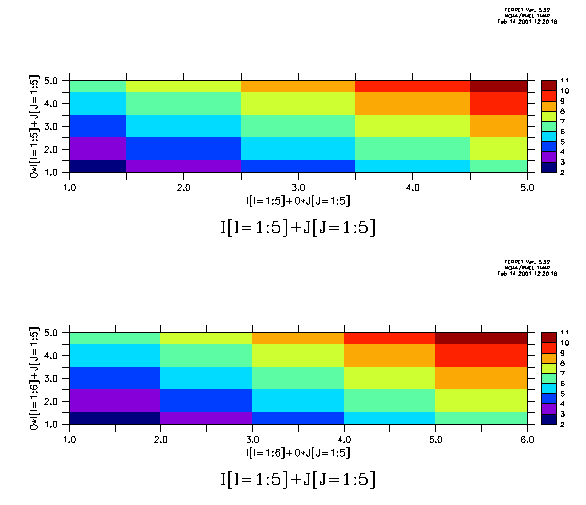# Cell edges for 3-argument shade plots

## Questions:

1) How does ferret generate boundaries for the 3-argument shade command?

2) Can these boundaries instead be user specified?

## Example:

There are two ways of specifying the coordinate arguments: Note the different cell boundaries in the x direction.## Explanation:

```In the 3-argument syntax you specify

Say
nVx - is the size of the "x" dimension of the values argument
nCx - is the size of the "x" dimension of the coordinate arguments
```

If nCx = nVx then the xcoords argument is presumed to give the locations of the points in the values argument, and the boundaries between points are computed to be the midpoints.

However, if nCx = nVx + 1 then the xcoords argument is presumed to give the locations of the cell boundaries. The identical discussion would apply to the cell boundaries in the y direction.

For both ways of specifying the coordinate arguments, make sure that the size of the xcoord argument always matches the size of the ycoord argument.

## Method:

```The Ferret commands for the above plot:

yes? set view upper